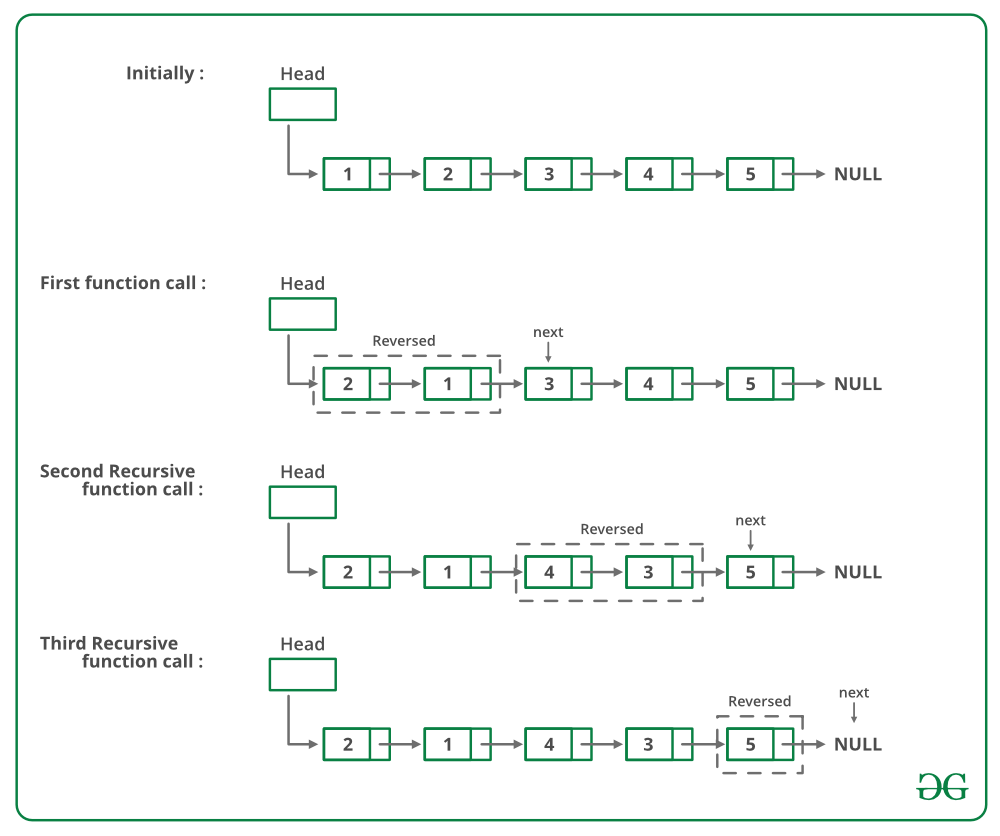Open In App

# Java Program For Pairwise Swapping Elements Of A Given Linked List

Given a singly linked list, write a function to swap elements pairwise.

```Input: 1->2->3->4->5->6->NULL
Output: 2->1->4->3->6->5->NULL

Input: 1->2->3->4->5->NULL
Output: 2->1->4->3->5->NULL

Input: 1->NULL
Output: 1->NULL```

For example, if the linked list is 1->2->3->4->5 then the function should change it to 2->1->4->3->5, and if the linked list is then the function should change it to.

METHOD 1 (Iterative):
Start from the head node and traverse the list. While traversing swap data of each node with its next node’s data.
Below is the implementation of the above approach:

## Java

 `// Java program to pairwise swap``// elements of a linked list``class` `LinkedList``{``    ``// Head of list``    ``Node head;` `    ``// Linked list Node``    ``class` `Node``    ``{``        ``int` `data;``        ``Node next;``        ``Node(``int` `d)``        ``{``            ``data = d;``            ``next = ``null``;``        ``}``    ``}` `    ``void` `pairWiseSwap()``    ``{``        ``Node temp = head;` `        ``/* Traverse only till there are``           ``atleast 2 nodes left */``        ``while` `(temp != ``null` `&&``               ``temp.next != ``null``)``        ``{``            ``// Swap the data``            ``int` `k = temp.data;``            ``temp.data = temp.next.data;``            ``temp.next.data = k;``            ``temp = temp.next.next;``        ``}``    ``}` `    ``// Utility functions``    ``/* Inserts a new Node at front of``       ``the list. */``    ``public` `void` `push(``int` `new_data)``    ``{``        ``/* 1 & 2: Allocate the Node &``                  ``Put in the data*/``        ``Node new_node = ``new` `Node(new_data);` `        ``// 3. Make next of new Node as head``        ``new_node.next = head;` `        ``// 4. Move the head to point to``        ``// new Node``        ``head = new_node;``    ``}` `    ``// Function to print linked list``    ``void` `printList()``    ``{``        ``Node temp = head;``        ``while` `(temp != ``null``)``        ``{``            ``System.out.print(temp.data + ``" "``);``            ``temp = temp.next;``        ``}``        ``System.out.println();``    ``}` `    ``// Driver code``    ``public` `static` `void` `main(String args[])``    ``{``        ``LinkedList llist = ``new` `LinkedList();` `        ``/* Created Linked List``           ``1->2->3->4->5 */``        ``llist.push(``5``);``        ``llist.push(``4``);``        ``llist.push(``3``);``        ``llist.push(``2``);``        ``llist.push(``1``);` `        ``System.out.println(``               ``"Linked List before calling pairWiseSwap() "``);``        ``llist.printList();` `        ``llist.pairWiseSwap();` `        ``System.out.println(``               ``"Linked List after calling pairWiseSwap() "``);``        ``llist.printList();``    ``}``}``// This code is contributed by Rajat Mishra`

Output:

```Linked list before calling pairWiseSwap()
1 2 3 4 5
2 1 4 3 5 ```

Time complexity: O(n), as we are traversing over the linked list of size N using a while loop.

Auxiliary Space: O(1), as we are not using any extra space.

METHOD 2 (Recursive):
If there are 2 or more than 2 nodes in Linked List then swap the first two nodes and recursively call for rest of the list.
Below image is a dry run of the above approach:Below is the implementation of the above approach:

## Java

 `/* Recursive function to pairwise swap``   ``elements of a linked list */``static` `void` `pairWiseSwap(node head)``{``    ``/* There must be at-least two nodes``       ``in the list */``    ``if` `(head != ``null` `&&``        ``head.next != ``null``)``    ``{``        ``/* Swap the node's data with data``           ``of next node */``        ``swap(head.data, head.next.data);` `        ``/* Call pairWiseSwap() for rest of``           ``the list */``        ``pairWiseSwap(head.next.next);``    ``}``}``// This code is contributed by aashish1995`

Time complexity: O(n), as we are traversing over the linked list of size N using recursion.

Auxiliary Space: O(1), as we are not using any extra space.
The solution provided there swaps data of nodes. If data contains many fields, there will be many swap operations. See this for an implementation that changes links rather than swapping data.

Please refer complete article on Pairwise swap elements of a given linked list for more details!